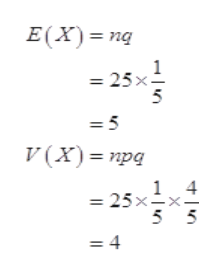# A store surveys customers to see if they are satisfied with the service they received. Samples of 25 surveys are taken.One in five people are unsatisfied. What is the variance of the mean of the sampling distribution of sample proportions forthe number of unsatisfied customers? What is the variance for satisfied customers?

Question
9 views

A store surveys customers to see if they are satisfied with the service they received. Samples of 25 surveys are taken.
One in five people are unsatisfied. What is the variance of the mean of the sampling distribution of sample proportions for
the number of unsatisfied customers? What is the variance for satisfied customers?

check_circle

Step 1

Form the given information,

Population proportions;

p = 1/5.

q = 1- p = 4/5.

Sample size(n) =25.

Step 2

Let X be number of unsatisfied customers in the sample.

X follow the binomial distribution with p =1/5 and n =25.

Thus,help_outlineImage TranscriptioncloseE(X) ng 1 = 25x = 5 V (X)= npq 4 1 = 25x=x. 5 5 =4 fullscreen
Step 3

### Want to see the full answer?

See Solution

#### Want to see this answer and more?

Solutions are written by subject experts who are available 24/7. Questions are typically answered within 1 hour.*

See Solution
*Response times may vary by subject and question.
Tagged in

### Statistics